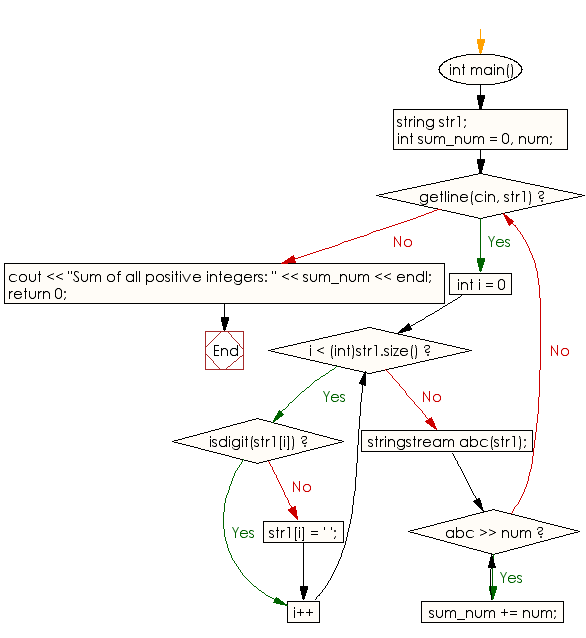﻿ C++ Exercises: Sum of all positive integers in a sentence - w3resource

# C++ Exercises: Sum of all positive integers in a sentence

## C++ Basic: Exercise-78 with Solution

Write a C++ program to sum all positive integers in a sentence.
Sample string: There are 12 chairs, 15 desks, 1 blackboard and 2 fans.
Output: 30

Sample Solution:

C++ Code :

``````#include <bits/stdc++.h>
using namespace std;

int main()
{
string str1;
int sum_num = 0, num;

while (getline(cin, str1)) {
for (int i = 0; i < (int)str1.size(); i++) {
if (isdigit(str1[i])) continue;
else {
str1[i] = ' ';
}
}

stringstream abc(str1);
while (abc >> num) {
sum_num += num;
}
}
cout << "Sum of all positive integers: " << sum_num << endl;
return 0;
}
``````

Sample Output:

```Input number: 12 chairs, 15 desks, 1 blackboard and 2 fans
Sum of all positive integers: 30
```

Flowchart:C++ Code Editor:

What is the difficulty level of this exercise?

﻿

## C++ Programming: Tips of the Day

Why is there no std::stou?

The most pat answer would be that the C library has no corresponding "strtou", and the C++11 string functions are all just thinly veiled wrappers around the C library functions: The std::sto* functions mirror strto*, and the std::to_string functions use sprintf.

Ref: https://bit.ly/3wtz2qA

We are closing our Disqus commenting system for some maintenanace issues. You may write to us at reach[at]yahoo[dot]com or visit us at Facebook